About once a month I see a question on the SAS Support Communities that involves what I like to call "computations with combinations." A typical question asks how to find k values (from a set of p values) that maximize or minimize some function, such as "I have 5 variables, and for each observation I want to find the largest product among any 3 values."

These types of problems are specific examples of a single abstract problem, as follows:

1. From a set of p values, generate all subsets that contain k < p elements. Call the subsets Y1, Y2, ..., Yt, where t equals "p choose k". (In SAS, use the COMB function to compute the number of combinations: t = comb(p,k).)
2. For each subset, evaluate some function on the subset. Call the values z1, z2, ..., zt.
3. Return some statistic of the zi. Often the statistics is a maximum or minimum, but it could also be a mean, variance, or percentile.

This is an "exhaustive" method that explicitly generates all subsets, so clearly this technique is impractical for large values of p. The examples that I've seen on discussion forums often use p ≤ 10 and small values of k (often 2, 3, or 4). For parameters in this range, an exhaustive solution is feasible.

This general problem includes "leave-one-out" or jackknife estimates as a special case (k = p – 1), so clearly this formulation is both general and powerful. This formulation also includes the knapsack problem in discrete optimization. In the knapsack problem, you have p items and a knapsack that can hold k items. You want to choose the items so that the knapsack holds as much value as possible. The knapsack problem maximizes the sum of the values whereas the general problem in this article can handle nonlinear functions of the values.

### Example data

You can use the following DATA set to simulate integer data with a specified number of columns and rows. I use the relatively new "Integer" distribution to generate uniformly distributed integers in the range [-3, 9].

```%let p = 5; /* number of variables */ %let NObs = 6; /* number of observations */ data have(drop=i j); call streaminit(123); array x[&p]; do i = 1 to &NObs; do j = 1 to dim(x); x[j] = rand("Integer", -3, 9); /* SAS 9.4M4 */ end; output; end; ;   proc print data=have; run;```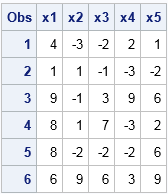### Computing with combinations in SAS/IML

For p = 5 and k = 3, the problem is: "For each observation of the 5 variables, find the largest product among any 3 values." In the SAS/IML language, you can solve problems like this by using the ALLCOMB function to generate all combinations of size k from the index set {1,2,...,p}. These values are indices that you can use to reference each combination of values. You can evaluate your function on each combination and then compute the max, min, mean, etc. For example, the following SAS/IML statements generate all combinations of 3 values from the set {1, 2, 3, 4, 5}:

```proc iml; p = 5; k = 3; c = allcomb(p, k); /* combinations of p items taken k at a time */ print c;```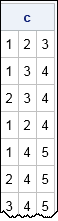A cool feature of the SAS/IML language is that you can use these values as column subscripts! In particular, the expression X[i, c] generates all 3-fold combinations of values in the i_th row. You can then use the SHAPE function to reshape the values into a matrix that has 3 columns, as follows:

```/* Example: find all combination of elements in the first row */ varNames = "x1":"x5"; use have; read all var varNames into X; close; Y = X[1, c]; /* all combinations of columns for 1st row */ M = shape(Y, nrow(c), k); /* reshape so each row has k elements */ prod = M[, #]; /* product of elements across columns */ print M prod;```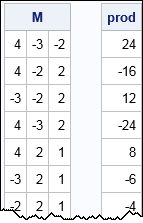Notice that each row of the matrix M contains k = 3 elements of Y. There are "5 choose 3" = 10 possible ways to choose 3 items from a set of 5, so the M matrix has 10 rows. Notice that you can use a subscript reduction operator (#) to compute the product of elements for each combination of elements. The maximum three-value product for the first row of data is 24.

The following loop performs this computation for each observation. The result is a vector that contains the maximum three-value product of each row. The original data and the results are then displayed side by side:

```/* for each row and for X1-X4, find maximum product of three elements */ result = j(nrow(X), 1); do i = 1 to nrow(X); Y = X[i, c]; /* get i_th row and all combinations of coloumns */ M = shape(Y, nrow(c), k); /* reshape so each row has k elements */ result[i] = max( M[,#] ); /* max of product of rows */ end; print X[colname=varNames] result[L="maxMul"];```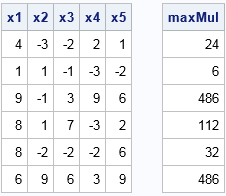Of course, if the computation for each observation is more complicated than in this example, you can define a function that computes the result and then call the module like this: result[i]= MyFunc(M);

### Generate all combinations in the DATA step

You can perform a similar computation in the DATA step, but it requires more loops. You can use the ALLCOMB function (or the LEXCOMBI function) to generate all k-fold combinations of the indices {1, 2, ..., p}. You should call the ALLCOMB function inside a loop from 1 to NCOMB(p, k). Inside the loop, you can evaluate the objective function on each combination of data values. Many DATA step functions such as MAX, MIN, SMALLEST, and LARGEST accept arrays of variables, so you probably want to store the variables and the indices in arrays. The following DATA step contains comments that describe each step of the program:

```%let p = 5; %let k = 3; %let NChooseK = %sysfunc(comb(&p,&k)); /* N choose k */ data Want(keep=x1-x&p maxProd); set have; array x[&p] x1-x&p; /* array of data */ array c[&k]; /* array of indices */ array r[&NChoosek]; /* array of results for each combination */ ncomb = comb(&p, &k); /* number of combinations */ do i=1 to &k; c[i]=0; end; /* zero the array of indices before first call to ALLCOMB */ do j = 1 to ncomb; call allcombi(&p, &k, of c[*]); /* generate j_th combination of indices */ /* evaluate function of the array {x[c], x[c], ..., x[c[k]]} */ r[j] = 1; /* initialize product to 1 */ do i=1 to &k; r[j] = r[j] * x[c[i]]; end; /* product of j_th combination */ end; maxProd = max(of r[*]); /* max of products */ output; run;   proc print data=Want; run;```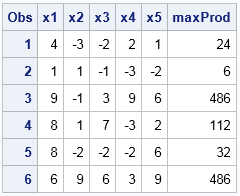The DATA step uses an array (R) of values to store the result of the function evaluated on each subset. For a MAX or MIN computation, this array is not necessary because you can keep track of the current MAX or MIN inside the loop over combinations. However, for more general problems (for example, find the median value), an array might be necessary.

In summary, this article shows how to solve a general class of problems. The general problem generates all subsets of size k from a set of size p. For each subset, you evaluate a function and produce a statistic. From among the "p choose k" statistics, you then choose the max, min, or some other measure. This article shows how to solve these problems efficiently in the SAS/IML language or in the SAS DATA step. Because this is a "brute force" technique, it is limited to small values of p. I suggest p ≤ 25.

The post Compute with combinations: Maximize a function over combinations of variables appeared first on The DO Loop.